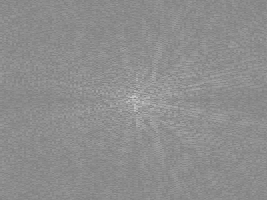## Sunday, April 22, 2007

### Extracting the magnitude component of an image Fourier transformNew result!

I finally succeeded in extracting the magnitude component of the image Fourier transform (shown at right).

Recapping the story so far

I previously created a picture of a bird, and a slightly translated version of the same image. I intend to use these images to test ideas about using the Fourier transform to automatically align pairs of images to create aligned stereoscopic pairs.

The input images, show in the previous post, are summarized below:

I took the plunge and learned to write a filter using the pbmplus environment (see previous post). Here is the program as I wrote and used it for this post:

The new PGM filter I made

I understand that it is tedious to mix GIMP and PBM tools in an image processing pipeline. Perhaps I will port the FFT image processing to PBM later...

What follows next is C language source code I just now wrote for a new image filter in the PBMPlus or NetPBM image processing tool kit:
/* pgm_fourier_recast.c - read a portable graymap produced by the** GIMP Fourier plug-in, and extract magnitude and phase components**** Copyright (C) 2007 by biospud@blogger.com**** Permission to use, copy, modify, and distribute this software and its** documentation for any purpose and without fee is hereby granted, provided** that the above copyright notice appear in all copies and that both that** copyright notice and this permission notice appear in supporting** documentation.  This software is provided "as is" without express or** implied warranty.*//*** 1) Place source file pgm_fourier_recast.c in directory with working build of netpbm/editor** 2) Add "pgm_fourier_recast" to list of files in Makefile** 3) "make pgm_fourier_recast" from netpbm/editor directory*/#include <stdio.h>#include <math.h>#include "pgm.h"typedef struct pgm_image_struct {int height;int width;gray maximumValue;gray** data;} PgmImage;PgmImage getInputImage( int argc, char *argv[] );PgmImage convertFourierToPhaseMagnitude(PgmImage inputImage);void writeImageAndQuit(PgmImage outputImage);double gimpFourierPixelToDouble(PgmImage image, int x, int y);double getNormalizationFactor(PgmImage image, int x, int y);gray doubleToGimpFourierPixel(double value, PgmImage image, int x, int y);int main( int argc, char *argv[] ){PgmImage inputImage;PgmImage outputImage;inputImage = getInputImage(argc, argv);outputImage = convertFourierToPhaseMagnitude(inputImage);writeImageAndQuit(outputImage);}PgmImage getInputImage( int argc, char *argv[] ) {const char* const usage = "[pgmfile]";int argn;FILE* inputFile;PgmImage answer;pgm_init( &argc, argv );argn = 1;if ( argn < argc ) {  inputFile = pm_openr( argv[argn] );  ++argn;} else {  inputFile = stdin;}if ( argn != argc )pm_usage( usage );answer.data = pgm_readpgm(    inputFile,    &answer.width,    &answer.height,    &answer.maximumValue    );pm_close( inputFile );return answer;}double gimpFourierPixelToDouble(PgmImage image, int x, int y) {/*** based on source code at** http://people.via.ecp.fr/~remi/soft/gimp/gimp_plugin_en.php3*/gray pixel = image.data[x][y];/*** renormalize** from (range 0 -> 255)** to range (-128 -> +127),*/double d128 = (double)(pixel) - 128.0; /* double128() */double bounded = (d128 / 128.0); /* unboost() */double unboosted0 = 160 * (bounded * bounded); /* unboost() */double unboosted = d128 > 0 ? unboosted0 : -unboosted0;  /* unboost() */double answer = unboosted / getNormalizationFactor(image, x, y);return answer;}/* Normalization factor that corrects scale of Fourier transform** pixel based upon distance from origin*/double getNormalizationFactor(PgmImage image, int x, int y) {/*** based on source code at** http://people.via.ecp.fr/~remi/soft/gimp/gimp_plugin_en.php3*/double cx = (double)abs(x - (image.width + 1)/2 + 1);double cy = (double)abs(y - (image.height + 1)/2 + 1);double energy = (sqrt(cx) + sqrt(cy));return energy*energy;}gray doubleToGimpFourierPixel(double value, PgmImage image, int x, int y) {double normalized = value * getNormalizationFactor(image, x, y);double bounded = fabs( normalized / 160.0 );double boosted0 = 128.0 * sqrt (bounded);double boosted = (value > 0) ? boosted0 : -boosted0;/*** renormalize** from range (-128 -> +127),** to (range 0 -> 255)*/int answer = (int)boosted + 128;if (answer >= 255) return 255;if (answer <= 0) return 0;return answer;}PgmImage convertFourierToPhaseMagnitude(PgmImage inputImage) {PgmImage answer;int outRows = inputImage.height;int outCols = inputImage.width;int row, col;double realDouble, imaginaryDouble;double magnitudeDouble, phaseDouble;gray realPixel, imaginaryPixel;gray magnitudePixel, phasePixel;int doUsePhase = 0;answer.height = outRows;answer.width = outCols;answer.maximumValue = inputImage.maximumValue;answer.data = pgm_allocarray( outCols, outRows );for ( row = 0; row < outRows; ++row ) {  for ( col = 0; col < outCols; col += 2) {        /* get pixel values from image */        realPixel = inputImage.data[row][col];        imaginaryPixel = inputImage.data[row][col + 1];        /* convert to doubles */        realDouble = gimpFourierPixelToDouble(inputImage, row, col);        imaginaryDouble = gimpFourierPixelToDouble(inputImage, row, col);        /* convert real/imaginary to magnitude/phase */        magnitudeDouble = sqrt(            realDouble * realDouble +            imaginaryDouble * imaginaryDouble            );        /* convert to pixel values */        magnitudePixel = doubleToGimpFourierPixel(            magnitudeDouble,            inputImage, row, col            );        if (doUsePhase) {            phaseDouble = atan2(imaginaryDouble, realDouble);            phasePixel = (int)(256.0 * phaseDouble / (2.0 * 3.14159));            while (phasePixel > 255) phasePixel -= 256;            while (phasePixel < 0) phasePixel += 256;        }        /*        i1 = inputImage.data[row][col];        v = gimpFourierPixelToDouble(inputImage, row, col);        i2 = doubleToGimpFourierPixel(v, inputImage, row, col);        printf("%.3g\t%.3g\t%.3g\t%.3g\n",            realDouble, imaginaryDouble, magnitudeDouble, phaseDouble);        */      answer.data[row][col] = magnitudePixel;        if (doUsePhase)          answer.data[row][col + 1] = phasePixel;        else          answer.data[row][col + 1] = magnitudePixel;    }}return answer;}void writeImageAndQuit(PgmImage outputImage) {/* Write resulting image */pgm_writepgm(    stdout,    outputImage.data,    outputImage.width,    outputImage.height,    outputImage.maximumValue,    0    );/* and clean up */pm_close( stdout );pgm_freearray(    outputImage.data,    outputImage.height    );exit( 0 );}
Original vs. translated images in Fourier magnitude space:

Phew! After writing this filter, I created the following "magnitude only" versions of the test images:

A superficial look suggests that the magnitude component is in fact very similar between the two images. But for automation, I need a quantitative measure to decide how similar two images are. More next time...